# 我用大数据分析了一线城市1000多份岗位招聘需求，告诉你如何科学找工作

### 软件设计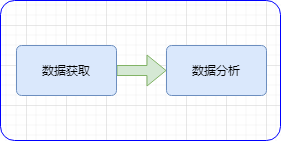### 详细实现

#### 数据获取

request库构造请求获取数据

cookie = s.cookies
text = req.json()


with open(os.path.join(self.path, '招聘_关键词_{}_城市_{}.csv'.format(self.keyword, self.city)), 			   'w',newline='', encoding='utf-8-sig') as f:
f_csv.writerows(data_list)


#### 数据分析

df_all.rename({'职位名称': 'position'}, axis=1, inplace=True) #axis=1代表index; axis=0代表column
df_all.rename({'详细链接': 'url'}, axis=1, inplace=True)
df_all.rename({'工作地点': 'region'}, axis=1, inplace=True)
df_all.rename({'薪资': 'salary'}, axis=1, inplace=True)
df_all.rename({'公司名称': 'company'}, axis=1, inplace=True)
df_all.rename({'经验要求': 'experience'}, axis=1, inplace=True)
df_all.rename({'学历': 'edu'}, axis=1, inplace=True)
df_all.rename({'福利': 'welfare'}, axis=1, inplace=True)
df_all.rename({'职位信息': 'detail'}, axis=1, inplace=True)
df_all.drop_duplicates(inplace=True)
df_all.index = range(df_all.shape)


from pyecharts.charts import Bar
regBar = Bar(init_opts=opts.InitOpts(width='1350px', height='750px'))
regBar.set_global_opts(title_opts=opts.TitleOpts(title="工作区域分布"),
toolbox_opts=opts.ToolboxOpts(),
visualmap_opts=opts.VisualMapOpts())

from pyecharts.commons.utils import JsCode
shBar = Bar(init_opts=opts.InitOpts(width='1350px', height='750px'))
shBar.set_series_opts(itemstyle_opts={
"normal": {
"color": JsCode("""new echarts.graphic.LinearGradient(0, 0, 0, 1, [{
offset: 0,
color: 'rgba(0, 244, 255, 1)'
}, {
offset: 1,
color: 'rgba(0, 77, 167, 1)'
}], false)"""),
}})
shBar.set_global_opts(title_opts=opts.TitleOpts(title="最高薪资范围分布"), toolbox_opts=opts.ToolboxOpts())

word_size_range=[20, 200], shape='diamond')
word.set_global_opts(title_opts=opts.TitleOpts(title="岗位技能关键词云图"),
toolbox_opts=opts.ToolboxOpts())


### 数据分析

#### 区域分布

C++岗位区域分布，北京 VS 深圳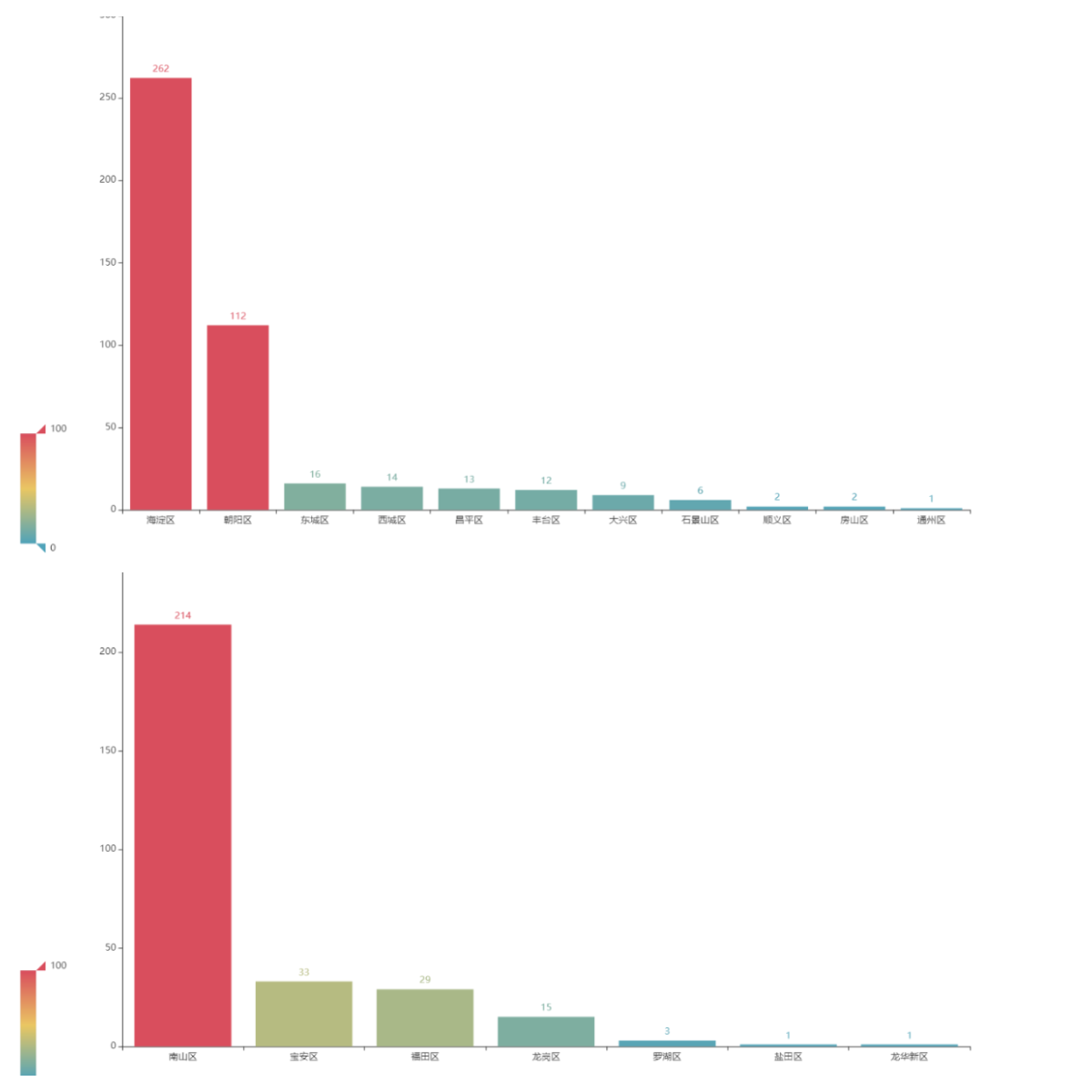#### 学历分布

C++岗位学历分布，北京 VS 深圳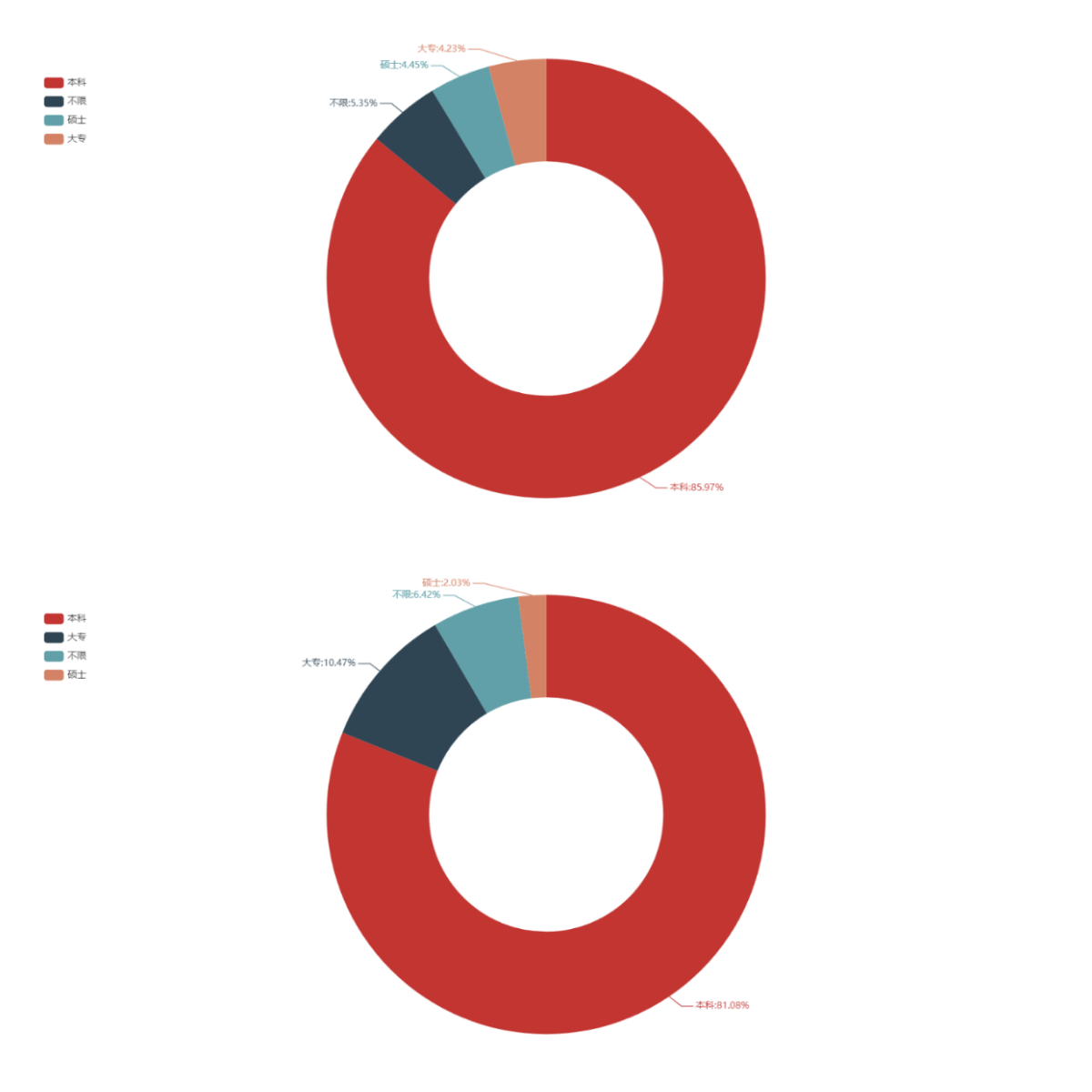#### 薪资分布

C++岗位薪资分布，薪资单位K。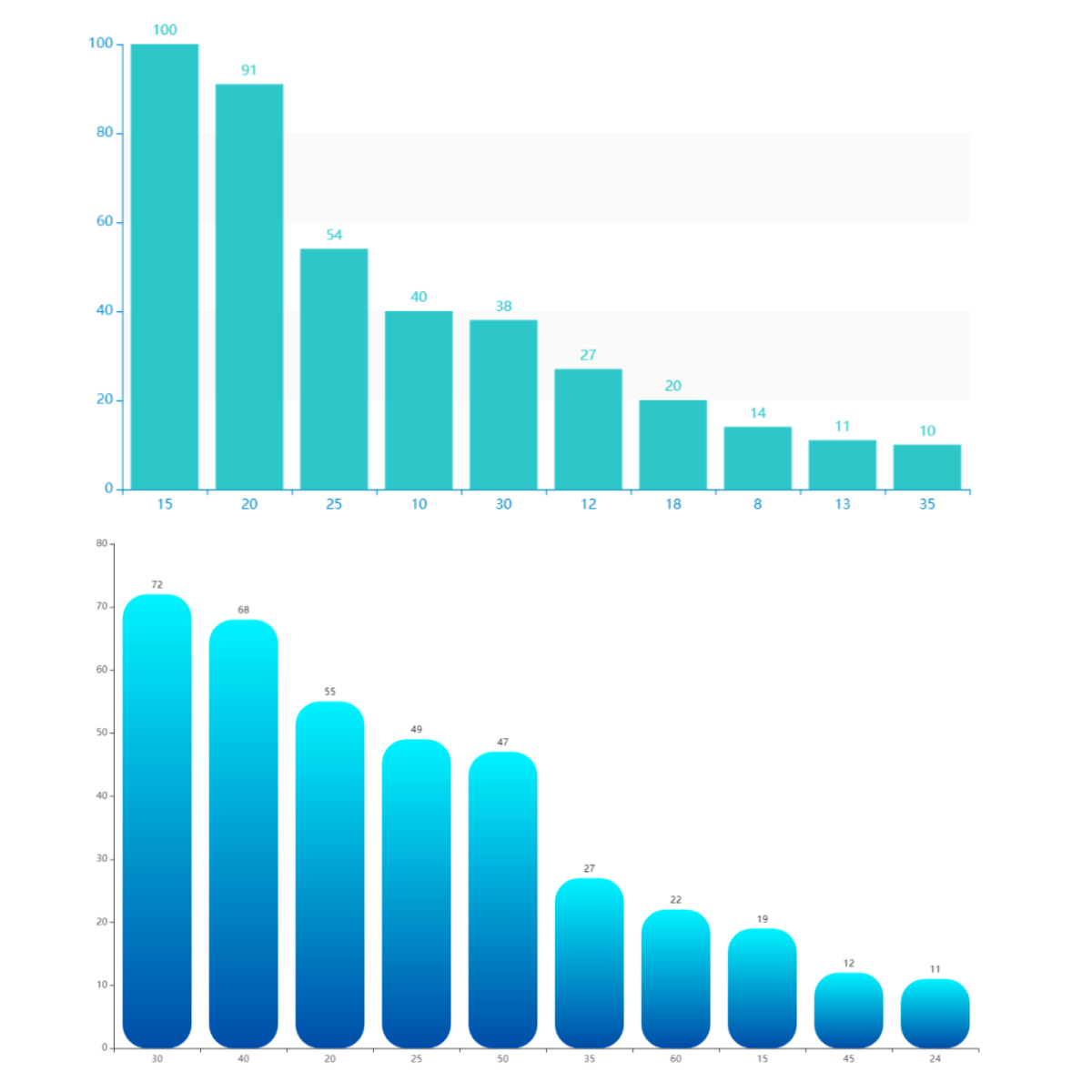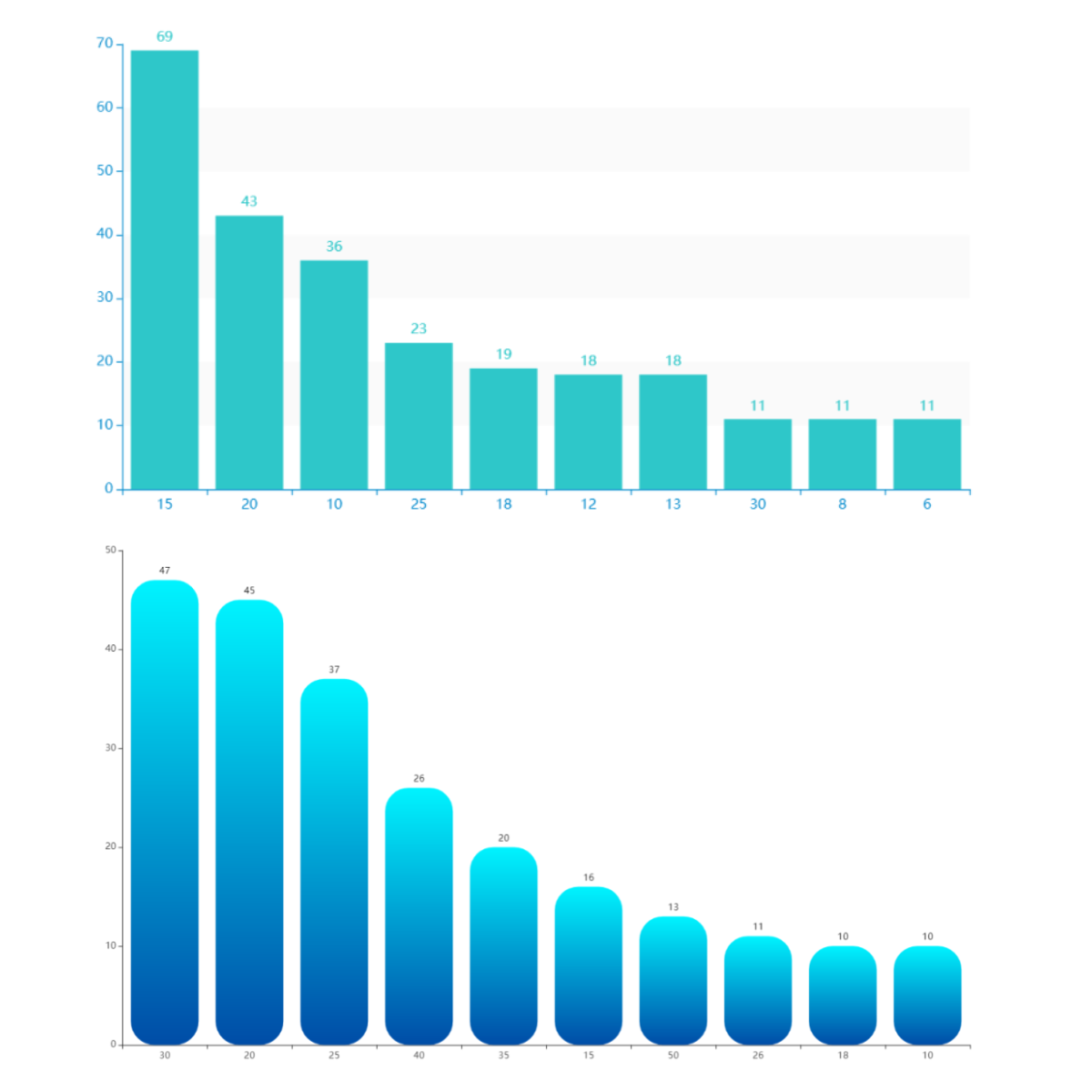#### 技能储备

C++岗位关键技能词云，北京 VS 深圳## 精彩文章推荐：

### 创作不易，点赞关注支持一下吧©️2020 CSDN 皮肤主题: 大白 设计师: CSDN官方博客点击重新获取扫码支付1.余额是钱包充值的虚拟货币，按照1:1的比例进行支付金额的抵扣。
2.余额无法直接购买下载，可以购买VIP、C币套餐、付费专栏及课程。余额充值